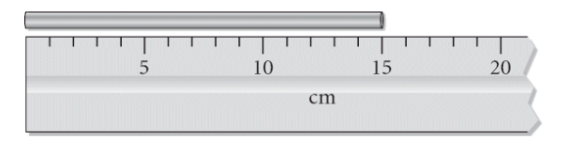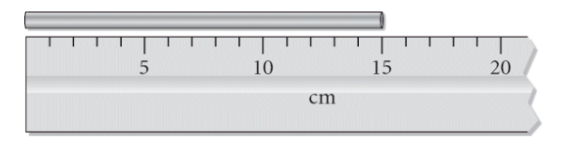# Problem: What is the length of the metal bar with the correct number of significant figures?a. 20 cmb. 15 cmc. 15.0 cmd. 15.00 cme. 15.000 cm

###### FREE Expert Solution

We’re being asked to determine the correct reading for the length of the metal bar with the correct number of significant figuresThe markings on the ruler correspond to 1 cm increments. We can see that the metal bar is just above the 15 cm mark.

80% (37 ratings)###### Problem Details

What is the length of the metal bar with the correct number of significant figures?a. 20 cm
b. 15 cm
c. 15.0 cm
d. 15.00 cm
e. 15.000 cm